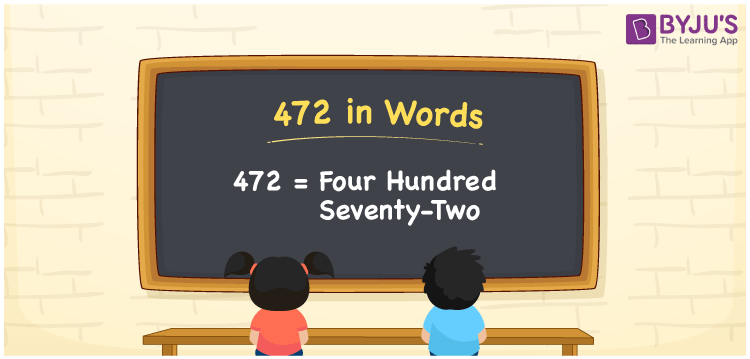# 472 in words

472 in words is written as Four Hundred and Seventy Two. 472 represents the count or value. The article on Counting Numbers can give you an idea about count or counting. The number 472 is used in expressions that relate to money, days, distance, length, weight and more. Let us consider an example for 472. “Distance between Chandigarh and Agra is more than Four Hundred and Seventy Two kilometers.” Another example, “There are Four Hundred and Seventy Two words in this article”.

 472 in words Four Hundred and Seventy Two Four Hundred and Seventy Two in Numbers 472

## 472 in English Words## How to Write 472 in Words?

We can convert 472 to words using a place value chart. The number 472 has 3 digits, so let’s make a chart that shows the place value up to 3 digits.

 Hundreds Tens Ones 4 7 2

Thus, we can write the expanded form as:

4 × Hundred + 7 × Ten + 2 × One

= 4 × 100 + 7 × 10 + 2 × 1

= 472

= Four Hundred and Seventy Two.

472 is the natural number that is succeeded by 471 and preceded by 473.

472 in words – Four Hundred and Seventy Two.

Is 472 an odd number? – No.

Is 472 an even number? – Yes.

Is 472 a perfect square number? – No.

Is 472 a perfect cube number? – No.

Is 472 a prime number? – No.

Is 472 a composite number? – Yes.

## Solved Example

1. Write the number 472 in expanded form

Solution: 4 × 100 + 7 × 10 + 2 × 1

We can write 472 = 400 + 70 + 2

= 4 × 100 + 7 × 10 + 2 × 1

## Frequently Asked Questions on 472 in words

Q1

### How to write 472 in words?

472 in words is written as Four Hundred and Seventy Two.
Q2

### State if True or False. 472 is divisible by 2?

True. 472 is divisible by 2.
Q3

### Is 472 a perfect square number?

No. 472 is not a perfect square number.